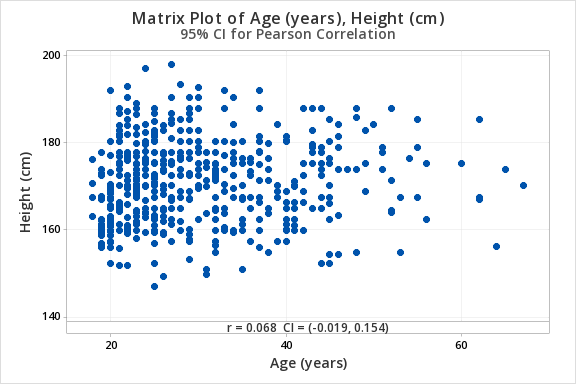# 12.2.1 - Hypothesis Testing

12.2.1 - Hypothesis Testing

In testing the statistical significance of the relationship between two quantitative variables we will use the five step hypothesis testing procedure:

1. Check assumptions and write hypotheses

In order to use Pearson's $$r$$ both variables must be quantitative and the relationship between $$x$$ and $$y$$ must be linear

Research Question Is the correlation in the population different from 0? Is the correlation in the population positive? Is the correlation in the population negative?
Null Hypothesis, $$H_{0}$$ $$\rho=0$$ $$\rho= 0$$ $$\rho = 0$$
Alternative Hypothesis, $$H_{a}$$ $$\rho \neq 0$$ $$\rho > 0$$ $$\rho< 0$$
Type of Hypothesis Test Two-tailed, non-directional Right-tailed, directional Left-tailed, directional
2. Calculate the test statistic

Minitab will not provide the test statistic for correlation. It will provide the sample statistic, $$r$$, along with the p-value (for step 3).

Optional: If you are conducting a test by hand, a $$t$$ test statistic is computed in step 2 using the following formula:

$$t=\dfrac{r- \rho_{0}}{\sqrt{\dfrac{1-r^2}{n-2}}}$$

In step 3, a $$t$$ distribution with $$df=n-2$$ is used to obtain the p-value.

3. Determine the p-value

Minitab will give you the p-value for a two-tailed test (i.e., $$H_a: \rho \neq 0$$). If you are conducting a one-tailed test you will need to divide the p-value in the output by 2.

4. Make a decision

If $$p \leq \alpha$$ reject the null hypothesis, there is evidence of a relationship in the population.

If $$p>\alpha$$ fail to reject the null hypothesis, there is not enough evidence of a relationship in the population.

5. State a "real world" conclusion.

Based on your decision in Step 4, write a conclusion in terms of the original research question.

# 12.2.1.1 - Example: Quiz & Exam Scores

12.2.1.1 - Example: Quiz & Exam Scores

## Example: Quiz and exam scores

Is there a relationship between students' quiz averages in a course and their final exam scores in the course?

Let's use the 5 step hypothesis testing procedure to address this process research question.

1. Check assumptions and write hypotheses

In order to use Pearson's $$r$$ both variables must be quantitative and the relationship between $$x$$ and $$y$$ must be linear. We can use Minitab to create the scatterplot using the file: Exam.mpx

Note that when creating the scatterplot it does not matter what you designate as the x or y axis. We get the following which shows a fairly linear relationship.

Our hypotheses:

• Null Hypothesis, $$H_{0}$$: $$\rho=0$$
• Alternative Hypothesis, $$H_{a}$$: $$\rho\ne0$$
2. Calculate the test statistic

Use Minitab to compute $$r$$ and the p-value.

1. Open the file in Minitab
2. Select Stat > Basic Statistics > Correlation
3. Enter the columns Quiz_Average and Final in the Variables box
4. Select the Results button and check the Pairwise correlation table in the new window
5. OK and OK
##### Pairwise Pearson Correlations
Sample 1 Sample 2 N Correlation 95% CI for $$\rho$$ P-Value
Final Quiz_Average 50 0.609 (0.398, 0.758) 0.000

Our sample statistic r = 0.609.

3. Determine the p-value

From our output the p-value is 0.000.

4. Make a decision

If $$p \leq \alpha$$ reject the null hypothesis, there is evidence of a relationship in the population.

5. State a "real world" conclusion.

There is evidence of a relationship between students' quiz averages and their final exam scores in the population.

# 12.2.1.2 - Example: Age & Height

12.2.1.2 - Example: Age & Height

Data concerning body measurements from 507 adults retrieved from body.dat.txt for more information see body.txt. In this example, we will use the variables of age (in years) and height (in centimeters) only.

For the full data set and descriptions see the original files:

For this example, you can use the following Minitab file: body.dat.mpx

Research question: Is there a relationship between age and height in adults?

1. Check assumptions and write hypotheses

Age (in years) and height (in centimeters) are both quantitative variables. From the scatterplot below we can see that the relationship is linear (or at least not non-linear).$$H_0: \rho = 0$$
$$H_a: \rho \neq 0$$

2. Calculate the test statistic

From Minitab:

##### Pairwise Pearson Correlations
Sample 1 Sample 2 N Correlation 95% CI for $$\rho$$ P-Value
Height (cm) Age (years) 507 0.068 (-0.019, 0.154) 0.127

$$r=0.068$$

3. Determine the p-value

$$p=.127$$

4. Make a decision

$$p > \alpha$$ therefore we fail to reject the null hypothesis.

5. State a "real world" conclusion.

There is not enough evidence of a relationship between age and height in the population from which this sample was drawn.

# 12.2.1.3 - Example: Temperature & Coffee Sales

12.2.1.3 - Example: Temperature & Coffee Sales

For this example, you can use the following Minitab file: cafedata.mpx

1. Check assumptions and write hypotheses

Maximum daily temperature and coffee sales are both quantitative variables. From the scatterplot below we can see that the relationship is linear.

$$H_0: \rho = 0$$
$$H_a: \rho \neq 0$$

2. Calculate the test statistic

From Minitab:

##### Pairwise Pearson Correlations
Sample 1 Sample 2 N Correlation 95% CI for $$\rho$$ P-Value
Max Daily Temperature (F) Coffees 47 -0.741 (-0.848, -0.577) 0.000

$$r=-0.741$$

3. Determine the p-value

$$p=.000$$

4. Make a decision

$$p \leq \alpha$$ therefore we reject the null hypothesis.

5. State a "real world" conclusion.

There is evidence of a relationship between the maximum daily temperature and coffee sales in the population.

  Link ↥ Has Tooltip/Popover Toggleable Visibility